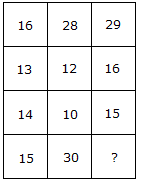# Verbal Reasoning - Character Puzzles - Discussion

### Discussion :: Character Puzzles - Character Puzzles 1 (Q.No.23)

23.

Which one will replace the question mark ?[A]. 60 [B]. 30 [C]. 20 [D]. 45

Explanation:

(16 + 13) = (14 + 15)

and (28 + 12) = (10 + 30)

Therefore (29 + 16) = (15 + 30).

 Ambika said: (Oct 15, 2015) 16+13 = 29-14 = 15. 28+12 = 40-10 = 30. 29+16 = 45-15 = 30.

 Santosh said: (May 17, 2016) Better explanation, Thankyou @Ambika.

 Sudhir said: (Sep 11, 2018) 17 11 19 12 13 16 25 4 ? Can anyone complete the matrix sequence?

 Rajesh said: (Feb 2, 2020) 13+1=14, 15+1=16. 10+2=12, 28+2=30. 15+1=16, 29+1=30.

 Dharu said: (Jul 25, 2020) 16+13-14=15. 28+12-10=30. 29+16-15=30.

 Ashish said: (Feb 3, 2021) Here the logic is; (16+13) - (14+15). (28+12) - (30+40). (29+16) - (15+30).